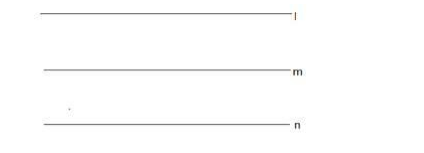# Prove that the line`
Question:

Prove that the line 12x – 5y – 3 = 0 is mid-parallel to the lines 12x – 5y + 7 = 0 and 12x – 5y – 13 = 0

Solution:

Given: parallel lines $12 x-5 y-3=0,12 x-5 y+7=0,12 x-5 y-13=0$

To Prove : line $12 x-5 y-3=0$ is mid-parallel to the lines $12 x-5 y+7=0$ and $12 x-5 y$ $-13=0$

Formula used: The distance between the parallel lines $a x+b y+c=0$ and $a x+b y+d$ $=0$ is,

$D=\frac{|d-c|}{\sqrt{a^{2}+b^{2}}}$

The equation of line 1 is $12 x-5 y+7=0$

The equation of line $m$ is $12 x-5 y-3=0$

The equation of line $n$ is $12 x-5 y-13=0$Let $D_{1}$ be the distance between the lines $I$ and $m$.

Here $a=12, b=-5, c=7, d=-3$

$D_{1}=\frac{|-3-7|}{\sqrt{12^{2}+(-5)^{2}}}=\frac{|-10|}{\sqrt{144+25}}=\frac{10}{\sqrt{169}}=\frac{10}{13}$

The distance between the parallel lines $I$ and $m$ is $\frac{10}{13}$ units

Let $D_{2}$ be the distance between the lines $m$ and $n$.

Here $a=12, b=-5, c=7, d=-3$

$D_{2}=\frac{|-13-(-3)|}{\sqrt{12^{2}+(-5)^{2}}}=\frac{|-13+3|}{\sqrt{144+25}}=\frac{|-10|}{\sqrt{169}}=\frac{10}{13}$

The distance between the parallel lines $\mathrm{m}$ and $\mathrm{n}$ is $\frac{10}{13}$ units

Distance between the parallel lines l and m = Distance between the parallel lines m and n

Thus the line $12 x-5 y-3=0$ is mid-parallel to the lines $12 x-5 y+7=0$ and $12 x-5 y-$ $13=0$